# 问题描述

## Problem Description

Daisy已经做过原来的汉诺塔问题和汉诺塔II，但碰到这个问题时，她想了很久都不能解决，现在请你帮助她。现在有N个圆盘，她至少多少次移动才能把这些圆盘从最左边移到最右边？

## Sample Input

``````1
3
12
``````

## Sample Output

``````2
26
531440
``````

# 问题分析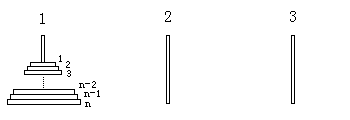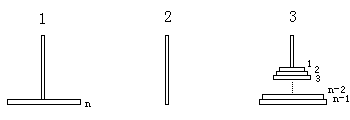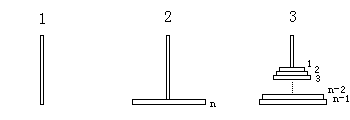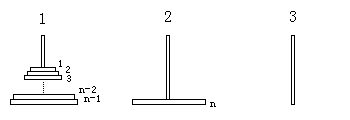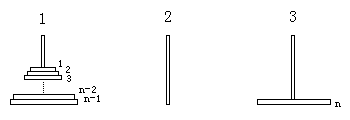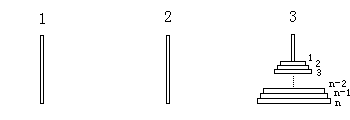``````∴ f(n) = 3 × f(n-1) + 2;
f(1) = 2;
``````

# 算法实现

``````f(n) = 3 × f(n-1) + 2
f(1) = 2
=>
f(n) + 1 = 3 × [f(n-1) + 1]
f(1) + 1 = 2 + 1 = 3
=>
f(n) + 1 = 3n
=>
f(n) = 3n - 1
``````

# 参考源码

redraiment使用Emacs Lisp批量迁移《HDU 2060-2069》